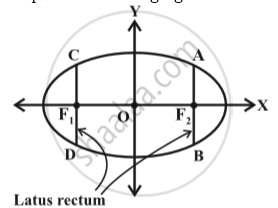Share

# Ellipse - Latus Rectum

#### description

• Latus Rectum in Ellipse

#### definition

Latus rectum of an ellipse is a line segment perpendicular to the major axis through any of the foci and whose end points lie on the ellipse in following fig.To find the length of the latus rectum of the ellipse x^2/a^2 + y^2/b^2 = 1
Let the length of AF_2 be l.
Then the coordinates of A are (c, l ),i.e., (ae, l )
Since A lies on the ellipse  x^2/a^2 + y^2/b^2 = 1,
(ae)^2/a^2+l^2/b^2=1

=> l^2 = b^2(1-e^2)

But e^2 = c^2/a^2 = (a^2 - b^2)/a^2 = 1- b^2/a^2

Therefore l^2 = b^4/a^2, i.e., l = b^2/a
Since the ellipse is symmetric with respect to y-axis ,AF_2 = F_2B and so length of the latus rectum is (2b)^2/a.

S